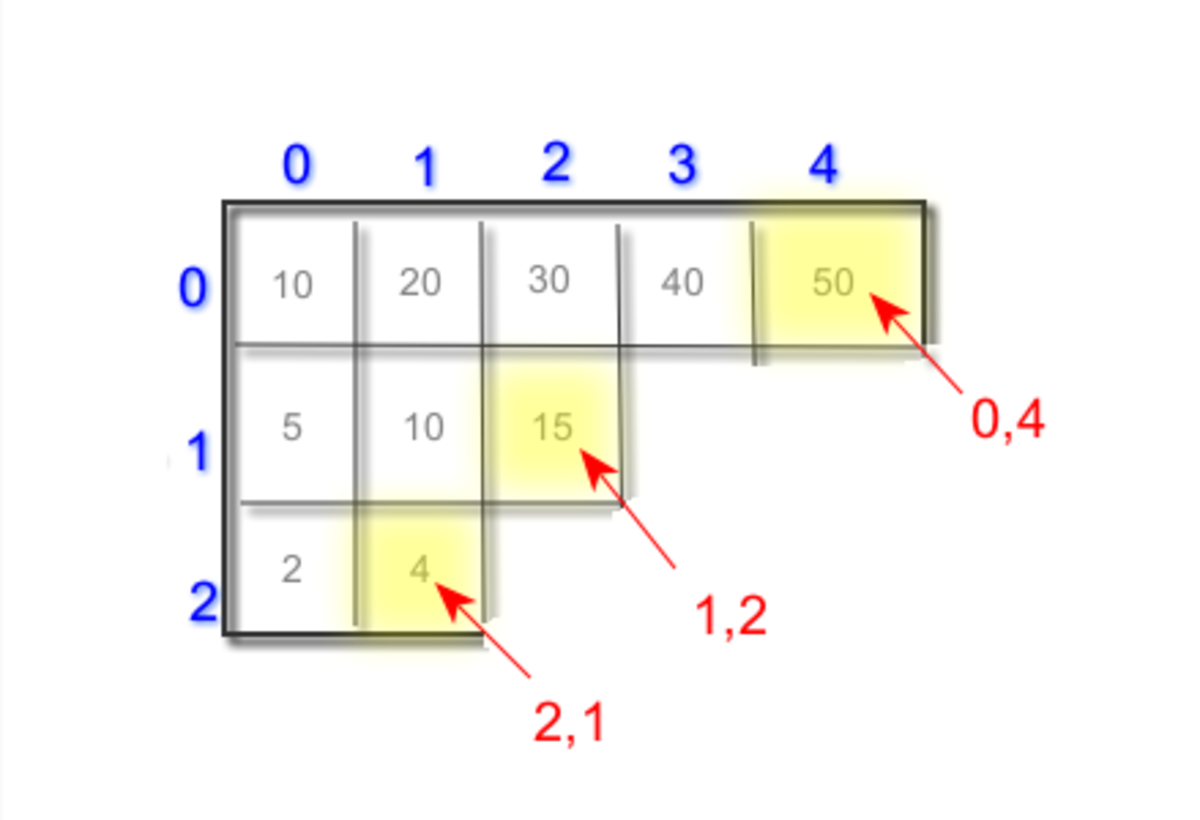# Java Examples: Ragged Array

• Author:
• Updated date:

I am a software engineer. I have been working with C++, MFC, and net technologies for 15 years. I like video games and reading books.

In Java, "ragged array" is an “array of array”. This means one can create an array in such a way that each element of the array is a reference to another array of same type. For example, a two-dimensional array of 3 x 2 refers to three rows and two columns (i.e. each row has two columns). We call this as “rectangular array”. In the case of a ragged array, the number of columns may not be fixed.

Note that "jagged array" and "ragged array" and "irregular dimensional array" all refers the same.

## 1. Declaring a Ragged Array

The below code (Listing 1) declares a ragged array:

## Listing 1

Here, ir_array is a ragged array and the dimension on the right-hand side [] specifies one dimension as fixed and another dimension as unknown. Here, in the above code we can call ir_array as One- dimensional array of double array. Like this one can create “multi-dimensional ragged array”.

## 2. Setting the Array References

Now, it is time to allocate space for three array of type double and setting its reference in the first dimension. The below code (Listing 2) shows setting up the ragged dimension:

## Listing 2

Here, we created three arrays to store double and the array size is 5,3,2. Each array is stacked in the array ir_array. For example, the first double array of size 5 is stored in the first slot of ir_array, and in the same way the second array of size 3 is stored in the second slot of the ir_array and so on.

In the above code, we had set up our ragged array. The three slots (let's say this as row) forms a three row and each row say represents an array. For example, ir_array is third row and represents an array of size 2, which represents the columns for it. OK, let us populate this array now.

## 3. Populating the Ragged Array

Have a look at the below code, which populates the ragged array:

## Listing 3

In the above code, the first array of double is filled with the value, which is multiples of 10 and the five element values are 10,20,30,40,50. The second array of double is filled with the values, which is multiple of 5. The third array is filled with the value, which is multiple of 2. After populating the array, the array will look as shown below:

Scroll to Continue

## 4. Picking Ragged Array Element

Once the ragged array is ready, the elements value can be picked just like a regular array. Here in our example, we set up a ragged array with two dimensions, and so to pick an element from this array, we need to locate the cell through its row and column. For example, let us consider ir_array and here ir_array tells first array of double with length 5 and second dimension  tells to locate the fifth element. Now consider the below code (Listing 4):

## Listing 4

In the above code example, we picked three elements from the jagged array and displayed that in the console output window. The elements picked are shown in the below picture:Picking an Element From Jagged ArrayAuthor

## 5. Dynamically Iterating All the Elements of Ragged Array

The length property of the array is useful to iterate the jagged array dynamically. Here in the below code ir_array denotes the “Array of Double Array” and its length is taken and iterated first. When we supply an index to ir-array, it represents the double array as in that index we store the reference of the double array. When we read length property (for example, ir_array.length,) it gives the length of the double array which is iterated as a nested loop.

In the below code snippet, we iterated through all the array elements and printed the result to the console output window:

## Listing 5

The complete example and its output is given below: